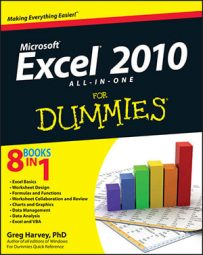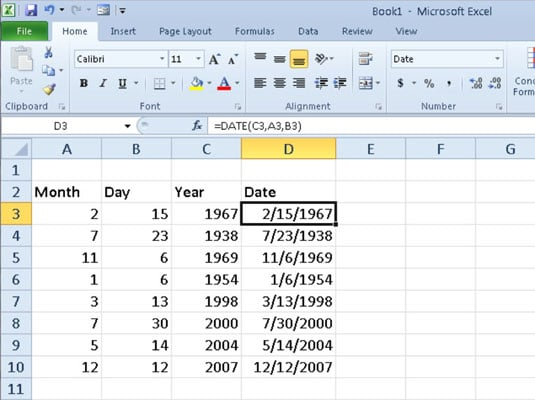##### Excel 2010 All-in-One For DummiesExcel 2010 contains a number of built-in date functions that you can use in your worksheets. Three common date functions are TODAY, DATE, and DATEVALUE, and they can come in very handy when you're trying to create a schedule in Excel.

## TODAY

The easiest and most commonly used date function has to be TODAY. This function takes no arguments and is always entered as follows:

`=TODAY()`

When you enter the TODAY function in a cell, Excel returns the current date by using the following Date format:

`7/23/2011`

Keep in mind that the date inserted into a cell with the TODAY function is not static. Whenever you open a worksheet that contains this function, Excel recalculates the function and updates its contents to the current date. This means that you don't usually use TODAY to input the current date when you're doing it for historical purposes (an invoice, for example) and never want it to change.

Excel stores dates as consecutive serial numbers, so you can use some mathematical operators with the TODAY function to designate dates in the past and future. For example, to show the date one week from the current date, you could use the formula =TODAY()+7.

## DATE

The DATE function returns a date serial number for the date specified by the year, month, and day arguments. This function uses the following syntax:

`=DATE(year,month,day)`

The DATE function comes in handy when you have a worksheet that contains the different parts of the date in separate columns, as shown in the following figure. You can use it to combine the three columns of date information into a single date cell that you can use in sorting and filtering.Using the DATE function to combine separate date information into a single entry.

## DATEVALUE

The DATEVALUE function returns the date serial number for a date that's been entered into the worksheet as text so that you can use it in date calculations. This function takes a single argument:

`=DATEVALUE(date_text)`

Suppose, for example, that you've made the following text entry in cell B12:

`'5/21/2004`

(Remember that when you preface an entry with an apostrophe, Excel inserts that entry as text even if the program would otherwise put it in as a value.) You can then convert this text entry into a date serial number by entering the following formula in cell C12 next door:

`=DATEVALUE(B12)`

Excel then returns the date serial number, 38128 to cell C12, which you can convert into a more intelligible date by formatting it with one of Excel's Date number formats.

You must convert the DATE and DATEVALUE functions into their calculated date serial numbers in order to sort and filter them. To convert these functions individually, select a cell, press F2 to activate Edit mode, and then press F9 to replace the function with the calculated date serial number; finally, click the Enter button on the Formula bar to insert this serial number into the cell.